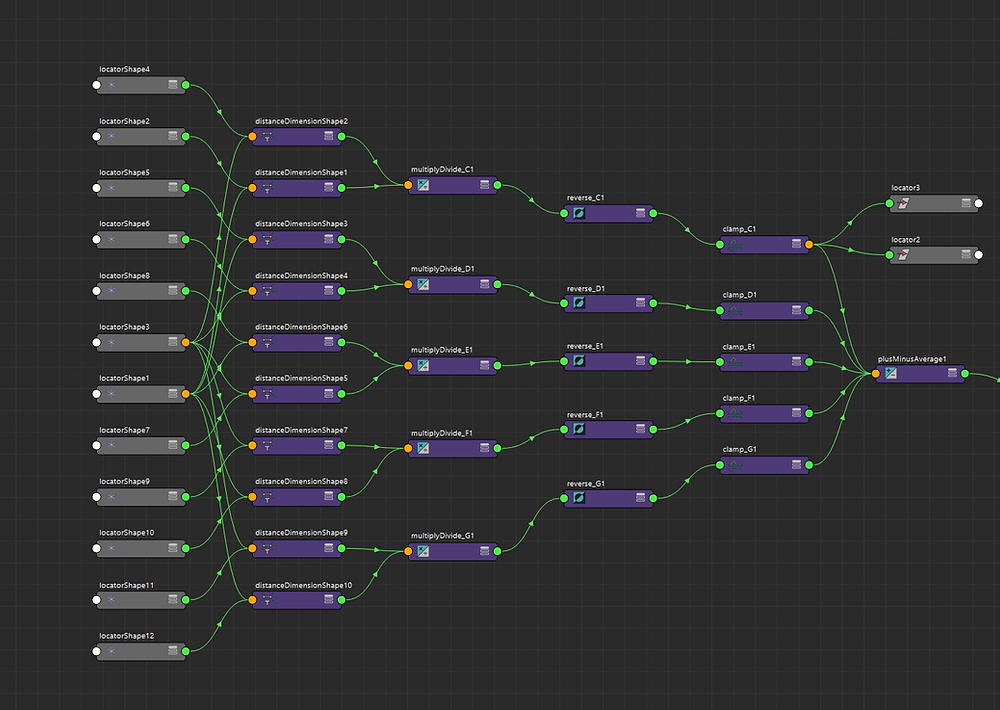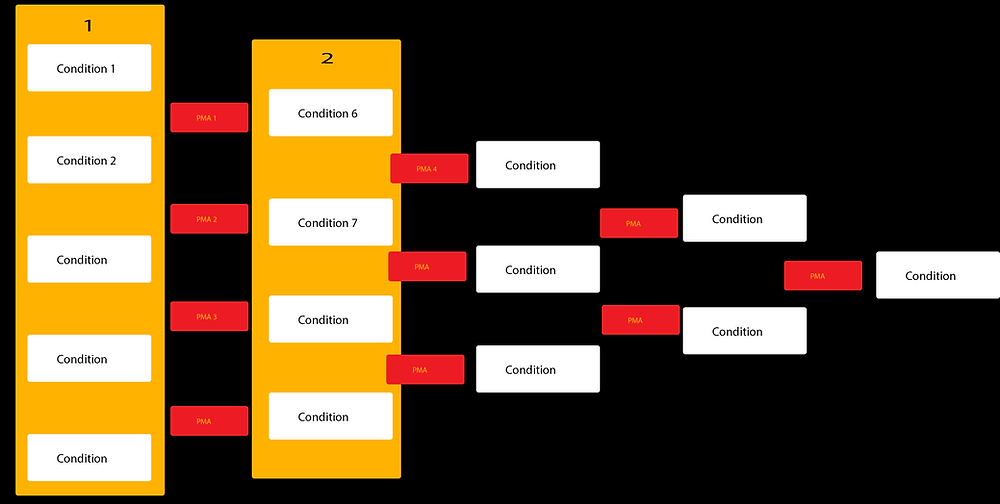•Liling Liu

# Learn how to interact with objects that change the color in Maya

Beat the five-note Xylophone and the stick changes the color from a touched object.The idea is beating the instrument and the stick will change the color. I created the xylophone mesh and put simple colors on it. The stick default color is light pink. When the stick touches the blue object, it will turn into blue color. Why I choose the xylophone, it is convenient to learn the children songs and interacts with objects.

For this case, I would like to present the children song that called lightly row. There have five lyrics on it. Lightly row song by numbers are 533 422, 1234555, 533 422, 13553, 2222234, 3333345, 533 422, 13551. ( C=1, D=2, E=3, F=4, G=5). If you would like to see more lyrics, you can go online to search for it.

Show Video URL: https://vimeo.com/298665701

## Calculate both of objects’ distanceUsing distance tool to measure two objects’ distance, so going to Create > Measure Tools > Distance Tool. Select the object and another target object to get the distance. Also, select the base object ( It can be a null object.) and another pose object to get the distance. Like the image, there are two distances to know pose, base, and target distances.

Put target locator into under the stick mesh and pose locaters into under the mesh. Independent on how many objects you have and will get more distances node. For my case, I have five objects, so I need to do fifth-time measurement and get the 10 distance dimensions.If you are not familiar with the utility nodes, you can go to AUTODESK KNOWLEDGE NETWORK

https://knowledge.autodesk.com/support/maya/learn-explore/caas/CloudHelp/cloudhelp/2017/ENU/Maya/files/GUID-DA9707D2-8A0D-4911-A010-8274C57D3FD3-htm.html

Adding a multiplyDivide node that two distance dimensions put in to get the current distance. Open multiplyDivide node to change the operation to Divide. MultiplyDivide output to reverse input. Reverse output to Clamp input, and giving limited Min number to 0, and Max to 1. Due to having many objects and distances, adding one plusMinusAverage node to put all together. Open the base locator attribute to add Distance Output attribute. PlusMinusAverage output to the base locator Distance Output.

## Condition the object’s distance to get the color

First of all, you should know how many condition nodes you should add it and compare each target object’s distance. Like previous, talked about how many distances you will get so that it likes the sum of the sequence number.For the formula the sum of sequence 1+2+3…+n=n(n+1)/2;

Proof is how to get n(n+1)/2: S=1+2+...+(n−1)+n. S=n+(n−1)+...+2+1, so 2S=(n+1)+(n+1)+...+(n+1)=n(n+1)

Divide by 2: S=n(n+1)2;

Above of formula the sum, the total of condition nodes called TCN = n(n+1)/2, n=object number.

Ex: N=1, TCN=1; N=2, TCN=3; N=3, TCN= 6...etc

In this case, I have five objects, so I need to add 15 condition nodes.Open node editor. Using a blendColor node to put the object’s color to Color 1 and Color 2 is default color. Each blendColor to connect to condition node to compare. If true, it will get the resulting color. If false, it appears the opposite resulting color.In the first column condition, blendcolor1 output put into Color if true, blendcolor2 output put into color if false, and Locator 1 (base) distance output put into condition1 first term. In the second column condition, condition1 output to color if true, and condition2 output to color if false. In this condition first term, using two target object distance to get it. Adding plusMinusAverage node, two target objects distances put into input3D and input3D. Open plusMinusAverage 1 node attribute, change operation to subtract. For plusMinusAverage5, using plusMinusAverage1 and plusMinusAverage2 output to input plusMinusAverage5 input3D and input3D and operation is subtract. Other nodes are the same processing, so repeating and get the result.384 次查看